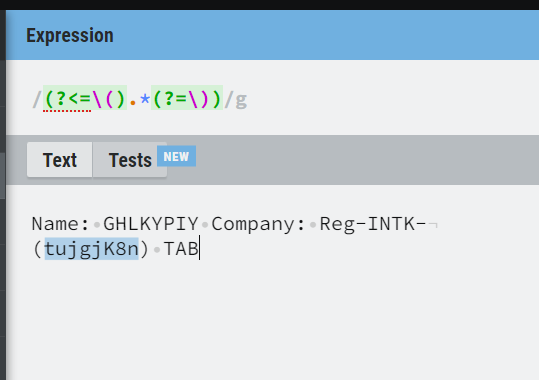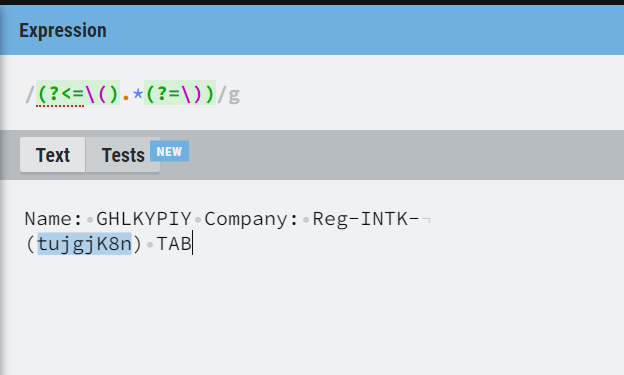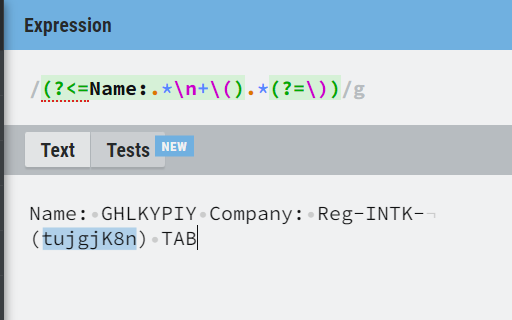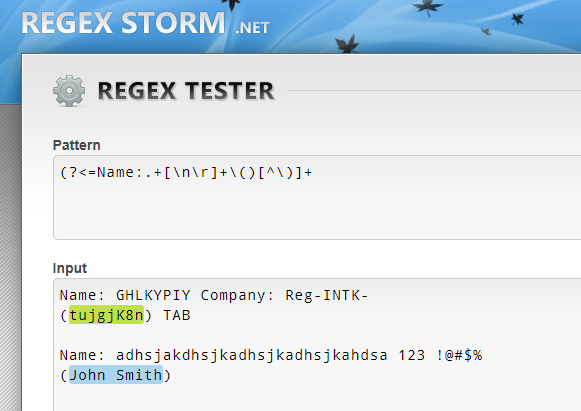# Regex Extaction in Bracket Value

I need help with the below scenario which is in brackets taking Name as Reference

Name: GHLKYPIY Company: Reg-INTK-
(tujgjK8n) TAB

`(?<=\().*(?=\))`Use the below regular expressions.

``````System.Text.RegularExpressions.Regex.Matches(yourstringinput.ToString,“((?<=\().*(?=\)))”)
``````Hope it helps!!

Hi Mahesh,

Thank you for the response it has multiple brackets in the pdf document but we need only the Name one as Reference

Thanks

@raghuram_katuri

``````(?<=Name: [A-Z]+\s+[A-Za-z]+.\s+[A-Za-z]+.[A-Z]+.\s+\().*(?=\)\s+[A-Z]+)
``````

or use the assign activity

``````System.Text.RegularExpressions.Regex.Matches(yourstringinput.ToString,"((?<=Name: [A-Z]+\s+[A-Za-z]+.\s+[A-Za-z]+.[A-Z]+.\s+\().*(?=\)\s+[A-Z]+))")
``````

Hope it helps!!

Okay @raghuram_katuri

Check the below one

``````System.Text.RegularExpressions.Regex.Matches(yourstringinput.ToString,“((?<=Name:.*\n+\().*(?=\)))”)
````````````System.Text.RegularExpressions.Regex.Matches(yourstringinput.ToString,“((?<=Name:\s+[A-Z]+\s+\w+\:\s+\w+\-\w+\-\n+\().*(?=\)))”)
``````

Hope it helps!!

Hello

Try this Regex Pattern:
(?<=Name:.+[\n\r]+\()[^\)]+

Left Assign:
str_Result

Right Assign:
System.Text.RegularExpressions.Regex.Match(yourString, (?<=Name:.+[\n\r]+\()[^\)]+).ToStringHopefully this helps:

Cheers

Steve

This topic was automatically closed 3 days after the last reply. New replies are no longer allowed.